Latest Banking jobs   »   NIACL AO QUANT

# Quantitative Aptitude Quiz For NIACL AO Prelims 2023 -03rd-September

Directions (1-5): Each question below is followed by two Statements [I] and [II]. You have to determine whether the data given in the statements are sufficient for answering the question. You should use the data and your knowledge of Mathematics to choose between the possible answers.
(a) if the Statement [I] alone is sufficient to answer the question but the Statement [II] alone is not sufficient
(b) if the Statement [II] alone is sufficient to answer the question but the Statement [I] alone is not sufficient
(c) if both Statement [I] and [II] together are needed to answer the question
(d) if either the Statement [I] alone or Statement [II] alone is sufficient to answer the question
(e) if you cannot get the answer from the Statements [I] and [II] together but need even more data

Q1. Ram sold an item. Find the M.P of the item?
[I]. Ram gave two successive discounts of 20% & 5% on marked price but after that take 25% more on discounted price as tax. Ram can earn 40 more if he sell the item at MP.
[II]. Ram gave two successive discount of MP i.e., 15% & 20% whereas Ram kept M.P. 50% more than the C.P. of that item.

Q2. Find the amount invested by Jagriti?
[I]. If jagriti invested half of the amount in Yes Bank at 5% p.a. for 3years and half the amount in Kotak Bank at 6% p.a. for 5 year, she got total Rs4500 as Simple Interest.
[II] . Jagriti will get 2420 more if she will invest in a bank at 10% p.a. for 3 year at compound interest rather than 10% p.a. for 2 year in same bank at compound interest

Q3. Whose body weight is second highest among the five boys Arun, Vinay, Suraj, Raju and Pratap?
I. Average weight of Arun, Suraj and Vinay is 68 kg and average weight of Raju and Pratap is 72 kg. Also Suraj is 78 kg. Raju is 68 kg and Vinay is 46 kg.
II. Average weight of Arun, Suraj, Vinay and Raju is 68 kg and also Suraj is 78 kg. Raju is 68 kg and Vinay is 46 kg. All of them have different weights.

Q4. What is the population of the city A?
I. The ratio of the population of males and females in city A is 27 : 23 and the difference between their population is 100000.
II. The population of city A is 80% of that of city B. The difference between populations of city A and city B is 312500.

Q5. How many students did participate in Singing?
I. The students who participated in dancing were 150% more than that who participated in Singing.
II. 150 students participated in dancing.

Q6. Manoj’s monthly salary is 25% more than Mohit’s salary. Mayank’s monthly salary is Rs 1750 more than Mohit’s monthly salary. Sum of Manoj’s, Mayank’s and Mohit’s yearly salary is Rs 3,33,000.
Quantity I: Sum of monthly salary of Manoj and Mohit together
Quantity II: Rs. 20,000
(a) Quantity I > Quantity II
(b) Quantity I < Quantity II
(c) Quantity I ≥ Quantity II
(d) Quantity I ≤ Quantity II
(e) Quantity I = Quantity II or No relation

Q7. A bag contains 8 white balls, 13 black balls and 5 green.
Quantity I: Probability that the first ball is white and the second ball is black if two balls are drawn at random from the bag one after another without replacement.
Quantity II: 6/35
(a) Quantity I > Quantity II
(b) Quantity I = Quantity II or No relation
(c) Quantity I ≥ Quantity II
(d) Quantity I < Quantity II
(e) Quantity I ≤ Quantity II

Q8. Quantity I: Profit share of ‘A’ out of total annual profit of Rs. 56,500. A, B and C enter into a partnership. ‘A’ invests Rs. 4000 for the whole year, ‘B’ puts in Rs. 6000 at the first and increasing to Rs. 8000 at the end of 4 months, whilst C puts in at first Rs. 8000 but withdraw Rs. 2000 at the end of 9 months.
Quantity II: Amount which when lend on C.I. at 20% interest being compounded annually for 3 years, gives total interest equal to Rs.9100
(a) Quantity I > Quantity II
(b) Quantity I ≥ Quantity II
(c) Quantity I < Quantity II
(d) Quantity I = Quantity II or No relation
(e) Quantity I ≤ Quantity II

Q9. 8 men and 4 women together can complete a piece of work in 6 days. Work done by a man in one day is double the work done by a woman in one day. 8 men and 4 women started working and after 2 days, 4 men left and 4 new women joined the work.
Quantity I: More days required to complete the work
Quantity II: 5 days
(a) Quantity I = Quantity II or No relation
(b) Quantity I ≥ Quantity II
(c) Quantity I < Quantity II
(d) Quantity I ≤ Quantity II
(e) Quantity I > Quantity II

Q10. The ratio of the age of Tina and Rakesh is 9 : 10 respectively. Ten years ago the ratio of their age was 4 : 5 respectively.
Quantity I: 22 years
Quantity II: Present age of Rakesh
(a) Quantity I > Quantity II
(b) Quantity I < Quantity II
(c) Quantity I = Quantity II or No relation
(d) Quantity I ≥ Quantity II
(e) Quantity I ≤ Quantity II

Solutions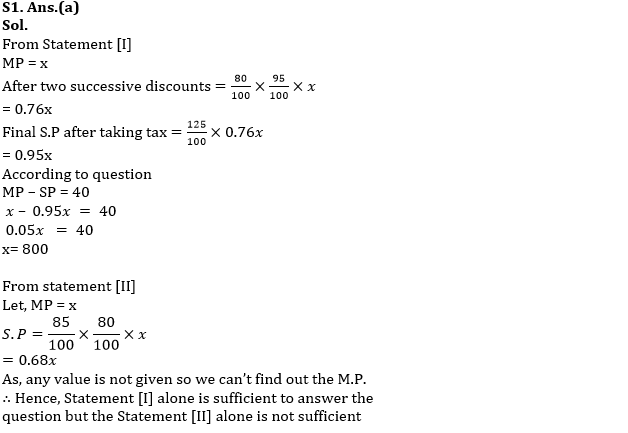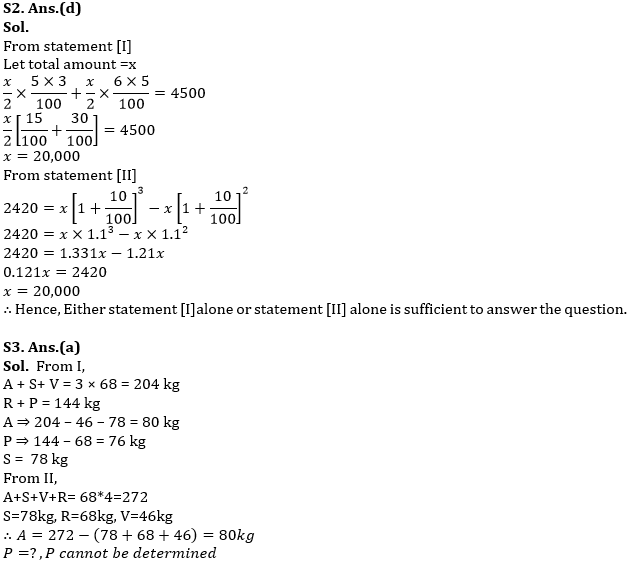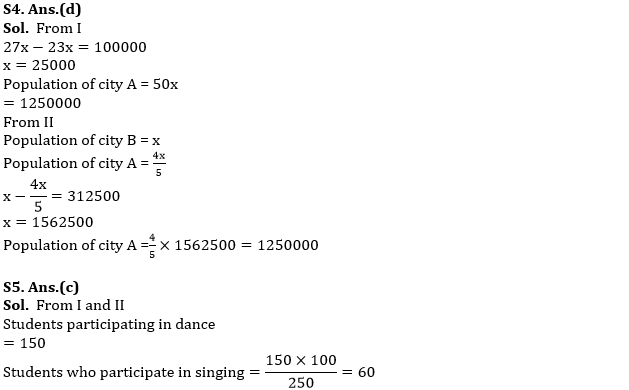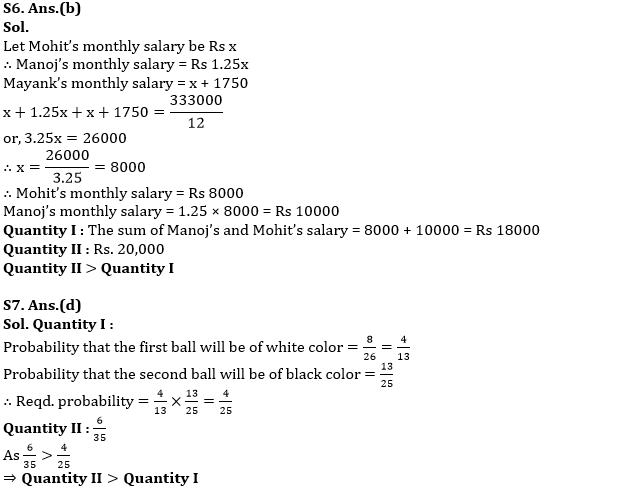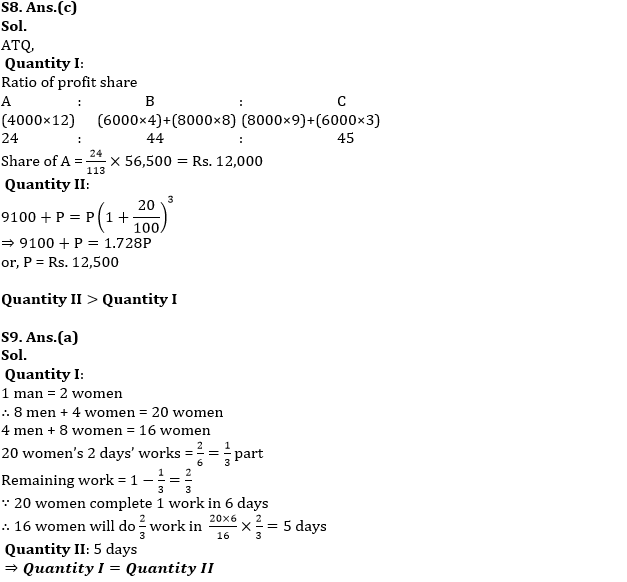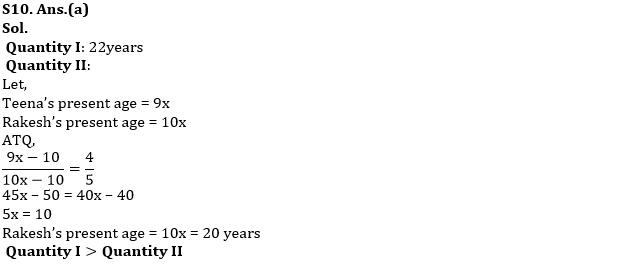## FAQs

### What are the subjects asked in NIACL AO Prelims exam 2023?

Subjects asked in NIACL AO Prelims are Reasoning Ability

#### Congratulations!Union Budget 2023-24: Free PDF